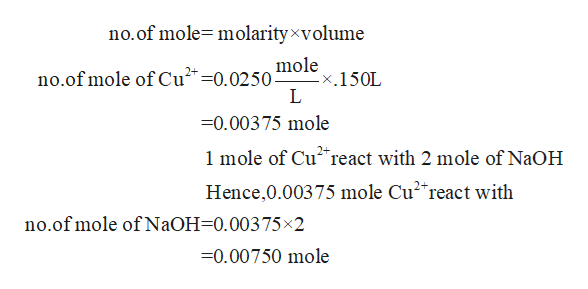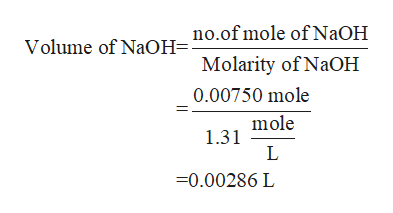# Let us assume that Cu(OH)2(s) is completely insoluble, which signifies that the precipitation reaction with NaOH(aq) (presented in the transition) would go to completion.Cu2+(aq)+2NaOH(aq) → Cu(OH)2(s)+2Na+(aq)If you had a 0.150 L solution containing 0.0250 M of Cu2+(aq), and you wished to add enough 1.31 M  NaOH(aq) to precipitate all of the metal, what is the minimum amount of the NaOH(aq) solution you would need to add? Assume that the NaOH(aq) solution is the only source of OH−(aq) for the precipitation.Express the volume to three significant figures and include the appropriate units.

Question
38 views

Let us assume that Cu(OH)2(s) is completely insoluble, which signifies that the precipitation reaction with NaOH(aq) (presented in the transition) would go to completion.

Cu2+(aq)+2NaOH(aq) → Cu(OH)2(s)+2Na+(aq)

If you had a 0.150 L solution containing 0.0250 M of Cu2+(aq), and you wished to add enough 1.31 M  NaOH(aq) to precipitate all of the metal, what is the minimum amount of the NaOH(aq) solution you would need to add? Assume that the NaOH(aq) solution is the only source of OH−(aq) for the precipitation.

Express the volume to three significant figures and include the appropriate units.
check_circle

Step 1

Given that,

Volume of Cu2+ is 0.150 L

Molarity of Cu2+ is 0.0250 M

Molarity of NaOH is 1.31 M

The given reaction is

Step 2

It means 1 mole of Cu2+ react with 2 mole of NaOH

Now, you calculate the no.of mole of Cu2+ and NaOHhelp_outlineImage Transcriptioncloseno.of mole molarityxvolume mole x.150L L no.of mole of Cu2-0.0250 -0.00375 mole 1 mole of Cu2 react with 2 mole of N2OH Hence,0.00375 mole Cu2 react with no.of mole of N2OH-0.00375x2 -0.00750 mole fullscreen
Step 3

Now, you calculate the...help_outlineImage Transcriptioncloseno.of mole of NaOH Volume of NaOH= Molarity of NaOH 0.00750 mole mole 1.31 L -0.00286 L fullscreen

### Want to see the full answer?

See Solution

#### Want to see this answer and more?

Solutions are written by subject experts who are available 24/7. Questions are typically answered within 1 hour.*

See Solution
*Response times may vary by subject and question.
Tagged in

### Chemistry